Name:    Chapter 9 Pre Test

Multiple Choice
Identify the choice that best completes the statement or answers the question.

1.

Find the principal square root of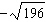, if possible. Do not use a calculator.
 a. 14 b.c.d.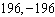e. no real roots

2.

Classify the numberas rational or irrational.
 a. rational b. irrational

3.

Classify the number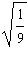as rational or irrational.
 a. rational b. irrational

4.

Evaluate the radical expression (if possible) without using a calculator.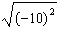a.b.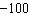c.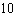d.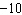e. not possible

5.

Evaluate the radical expression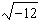without using a calculator. If not possible, state the reason.
 a.b.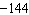c.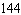d.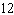e. not a real number

6.

Simplify the radical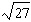, if possible.
 a.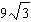b.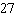c.d.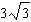e. cannot be simplified

7.

Simplify the radical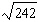, if possible.
 a.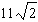b. 2 c.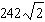d. 121 e. cannot be simplified

8.

The four corners are cut from a 3-foot-by- 6-foot sheet of plywood, as shown in the figure. Find the perimeter of the remaining piece of plywood. In the figure. Round your answer to two decimal places.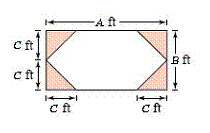a. 26.94 feet b. 10.24 feet c. 14.49 feet d. 21.94 feet e. 22.66 feet

9.

Multiply and simplify.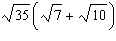a.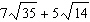b.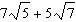c.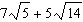d.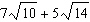e.10.

Multiply and simplify the expression.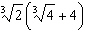a.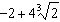b.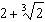c.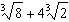d.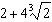e.11.

Multiply and simplify.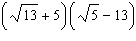a.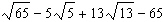b.c.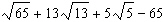d.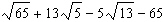e.12.

Complete the statement.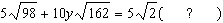a.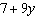b.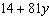c.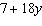d.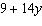e.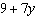13.

Solve, if possible.
 a.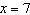b.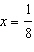c.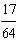d.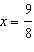e. no solution

14.

Use the Pythagorean Theorem to find the value of x. Round your answer to two decimal places.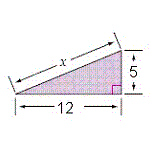a.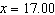b.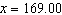c.d.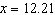e.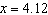15.

Subtract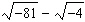and write in standard form.
 a.b.c.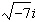d.e.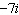16.

Determine the values of a and b that satisfy the equation.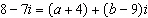a.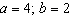b.c.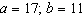d.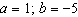e.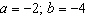17.

Perform the operation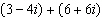and write the result in standard form.
 a.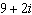b.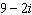c.d.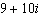e.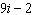18.

Perform the operation and write the result in standard form.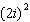a.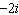b.c.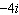d.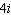e.19.

Multiply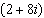by its complex conjugate and simplify.
 a. 68 b.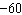c.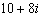d.e. 10

20.

Multiply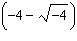by its complex conjugate and simplify.
 a. 20 b.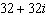c.d. 32 e.